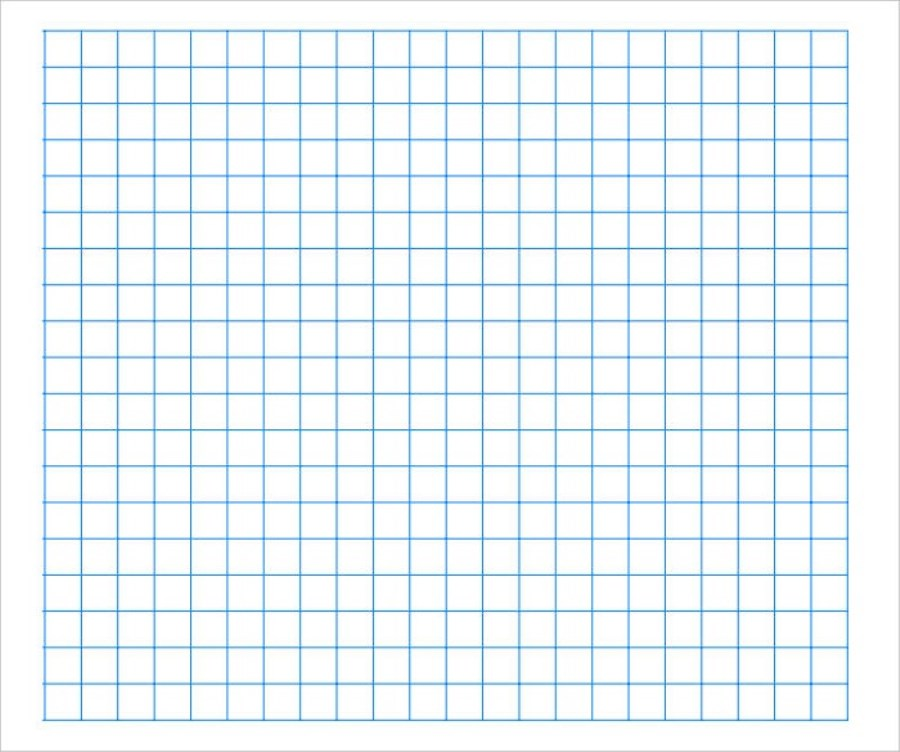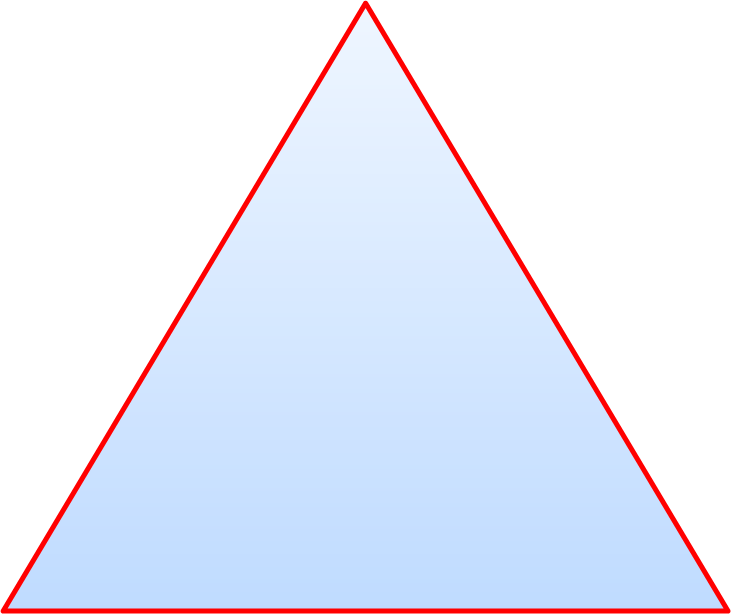The Equilateral Triangle

To construct a tetrahedron, you must first design and construct an equilateral triangle. There are two easy methods to do this task.
First, use a standard, horizontal sheet of graph paper, minimum (18cm vertically and 20cm wide), to design the triangle.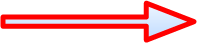20 cm, Base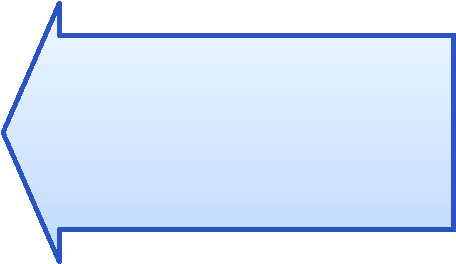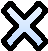17 cmA
B
C
D
Each angle in this equilateral triangle = 60° degrees
Start by drawing the base line (A,B)  20 cm long. Dont forget to start counting from 0. Draw the line accurately.
Next, starting from A, count over 10 cm and mark that point as D. This is the centre of line A B.  Count vertically from D, 17cm, and mark point C. Join C and D accurately. This is the altitude or height of your triangle.

The exact distance from D to C should be 17.32cm according to the Pythagorean theorem,  a very important mathematical calculation  you will study in the future.

Seventeen centimeters is close enough for your purpose. Your purpose is to design and construct a tetrahedron with a volume of one cubic decimeter.

You will then be able to visually compare a one cubic decimeter tetrahedron to your cubic decimeter cube.

Carefully join points A and C and points B and C.

Altitude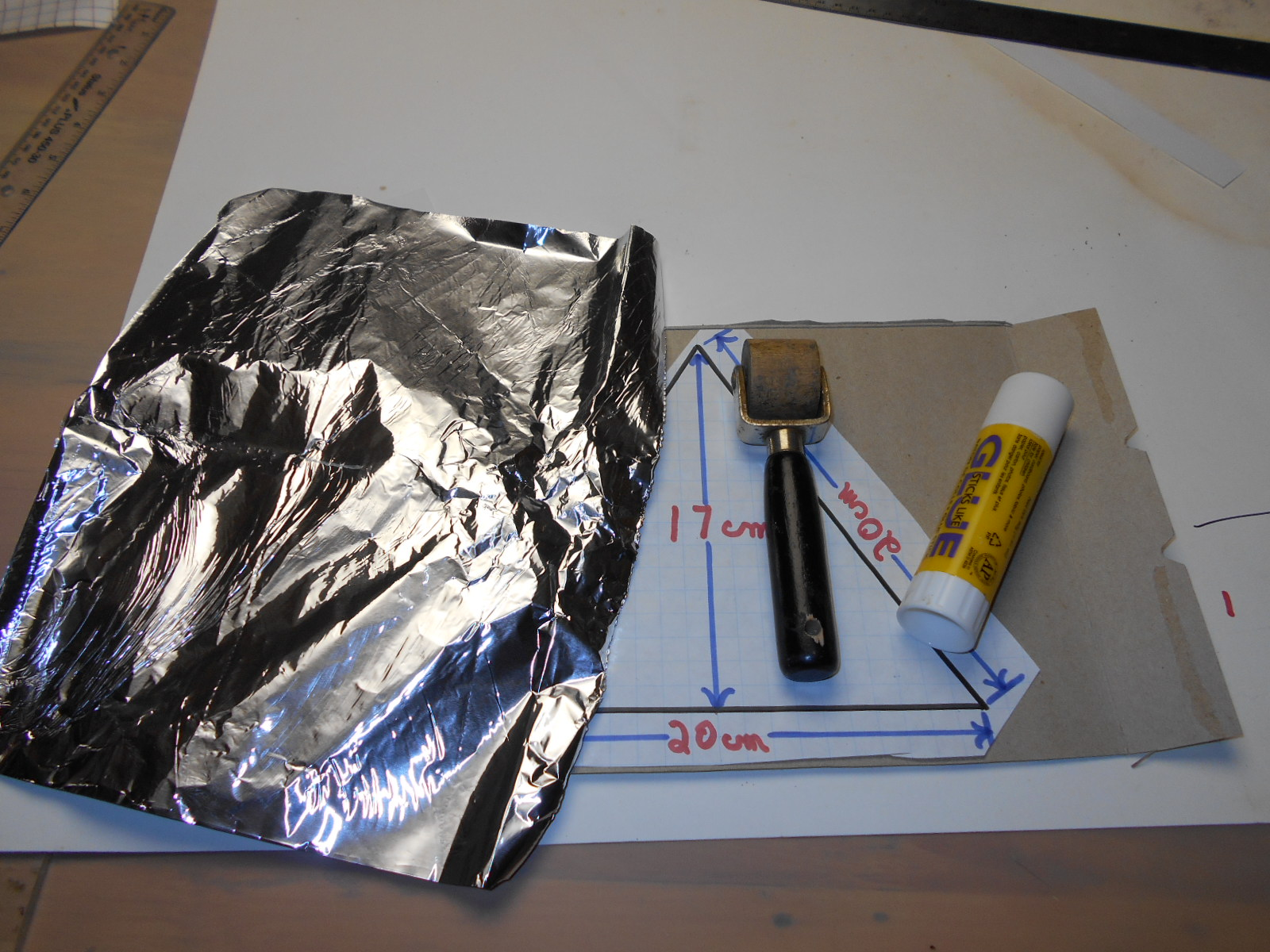After completing your design,  use a pair of scissors to rough cut the outside.

After completing your rough cut,  turn your  design over and apply a smooth amount of glue from your glue stick.

Find a piece of boxboard larger than your design. Carefully place the design with the glue side on top of the boxboard.

Using a roller and a material such as aluminum foil or thin plastic to protect your design and roller, roll the paper so that it is securely glued to the boxboard.

Carefully cut the equilateral triangle to size.

Now you  need to make a decision. You can use the equilateral triangle that you have made as a template.

Use that template to trace three more congruent triangles. Cut the equilateral triangles carefully. Now you have four congruent equilateral triangles ready to assemble and form a tetrahedron.

A second method is to search for a compass that can span a minimum of 20cm. With that compass you can make an equilaterial triangleRough Cut